S Coombes | Center for Mathematical Medicine | School of Mathematical Sciences | University of Nottingham

## Evans functions for integral neural field equations with Heaviside firing rate function

#### S Coombes and M R Owen

In this paper we show how to construct the Evans function for traveling wave solutions of integral neural field equations when the firing rate function is a Heaviside. This allows a discussion of wave stability and bifurcation as a function of system parameters, including the speed and strength of synaptic coupling and the speed of axonal signals. The theory is illustrated with the construction and stability analysis of front solutions to a scalar neural field model and a limiting case is shown to recover recent results of L. Zhang [On stability of traveling wave solutions in synaptically coupled neuronal networks, Differential and Integral Equations, 16, (2003), pp.513-536.]. Traveling fronts and pulses are considered in more general models possessing either a linear or piecewise constant recovery variable. We establish the stability of coexisting traveling fronts beyond a front bifurcation and consider parameter regimes that support two stable traveling fronts of different speed. Such fronts may be connected and depending on their relative speed the resulting region of activity can widen or contract. The conditions for the contracting case to lead to a pulse solution are established. The stability of pulses is obtained for a variety of examples, in each case confirming a previously conjectured stability result. Finally we show how this theory may be used to describe the dynamic instability of a standing pulse that arises in a model with slow recovery. Numerical simulations show that such an instability can lead to the shedding of a pair of traveling pulses.
Click on the picture to obtain a pdf version of the manuscript.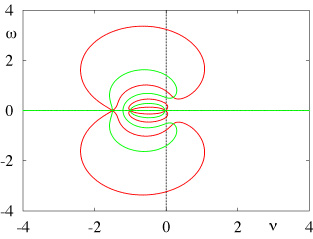Click on the pictures to see animations (in .mov format)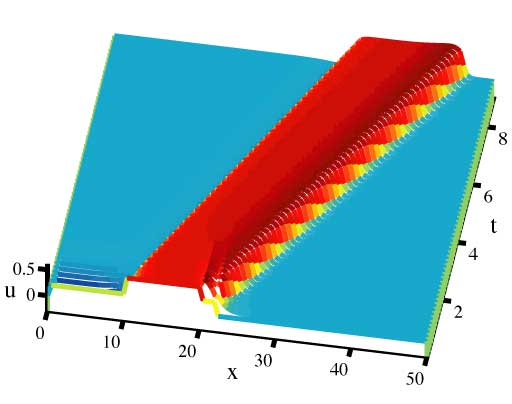Figure 4.4 (a). Reversing and forward propagating fronts giving rise to an expanding region of activity.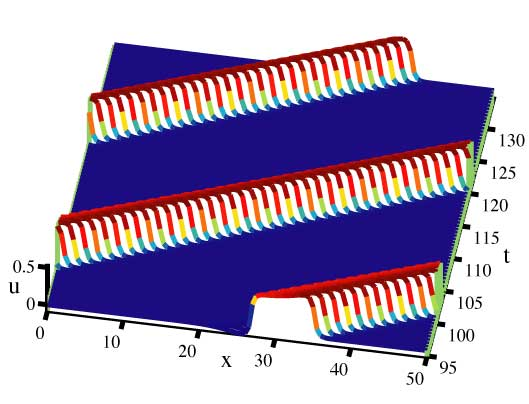Figure 4.4 (b,c). Reversing and forward propagating fronts converging onto a traveling pulse.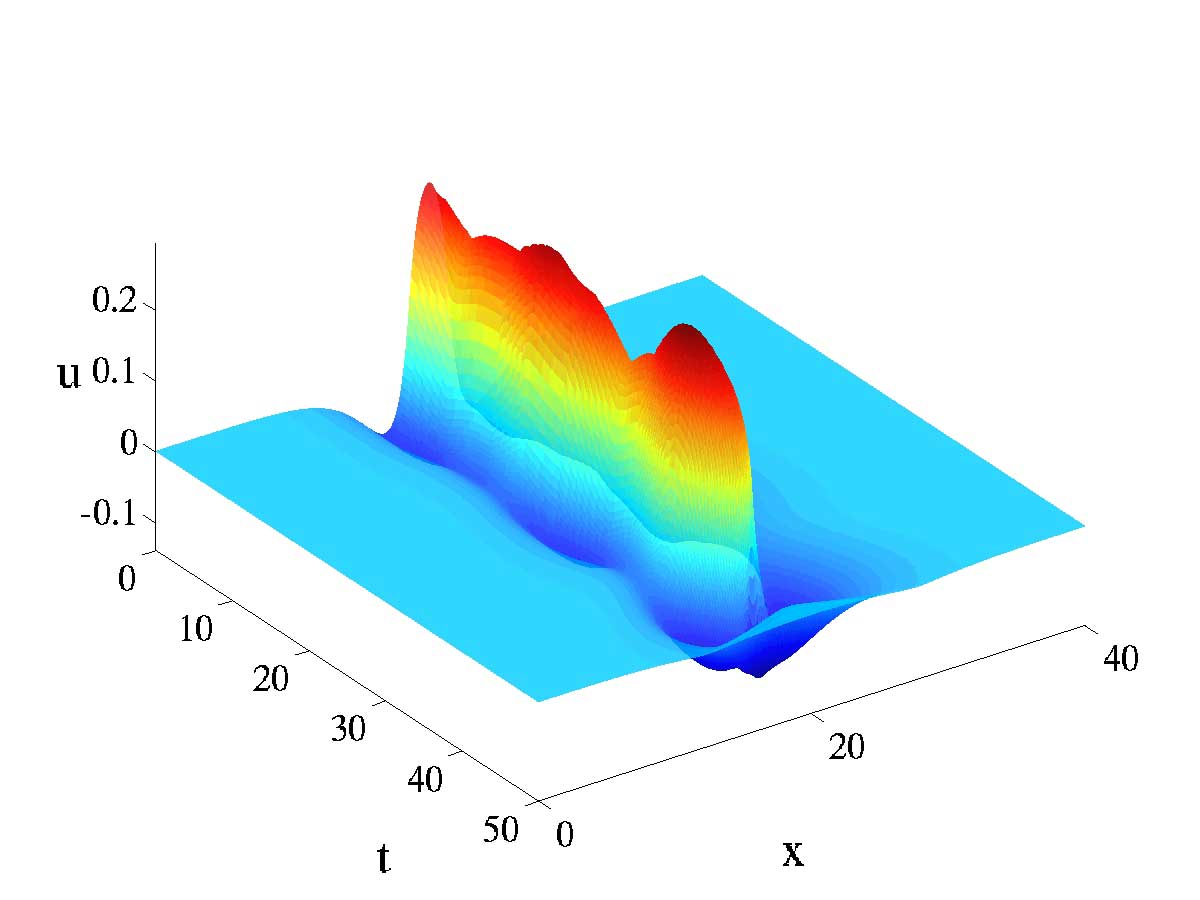Figure 5.5. A narrow bump dying.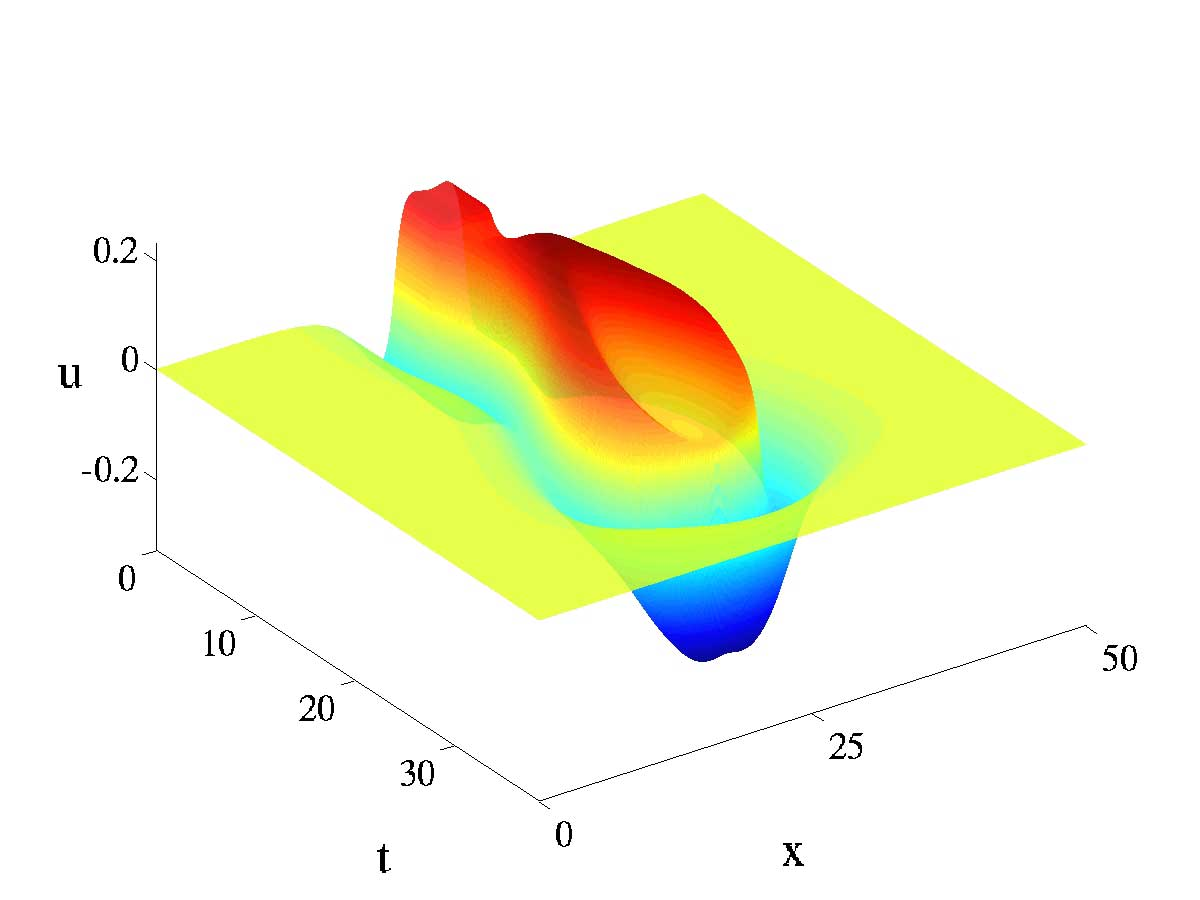Figure 5.7. A dimple bump dying.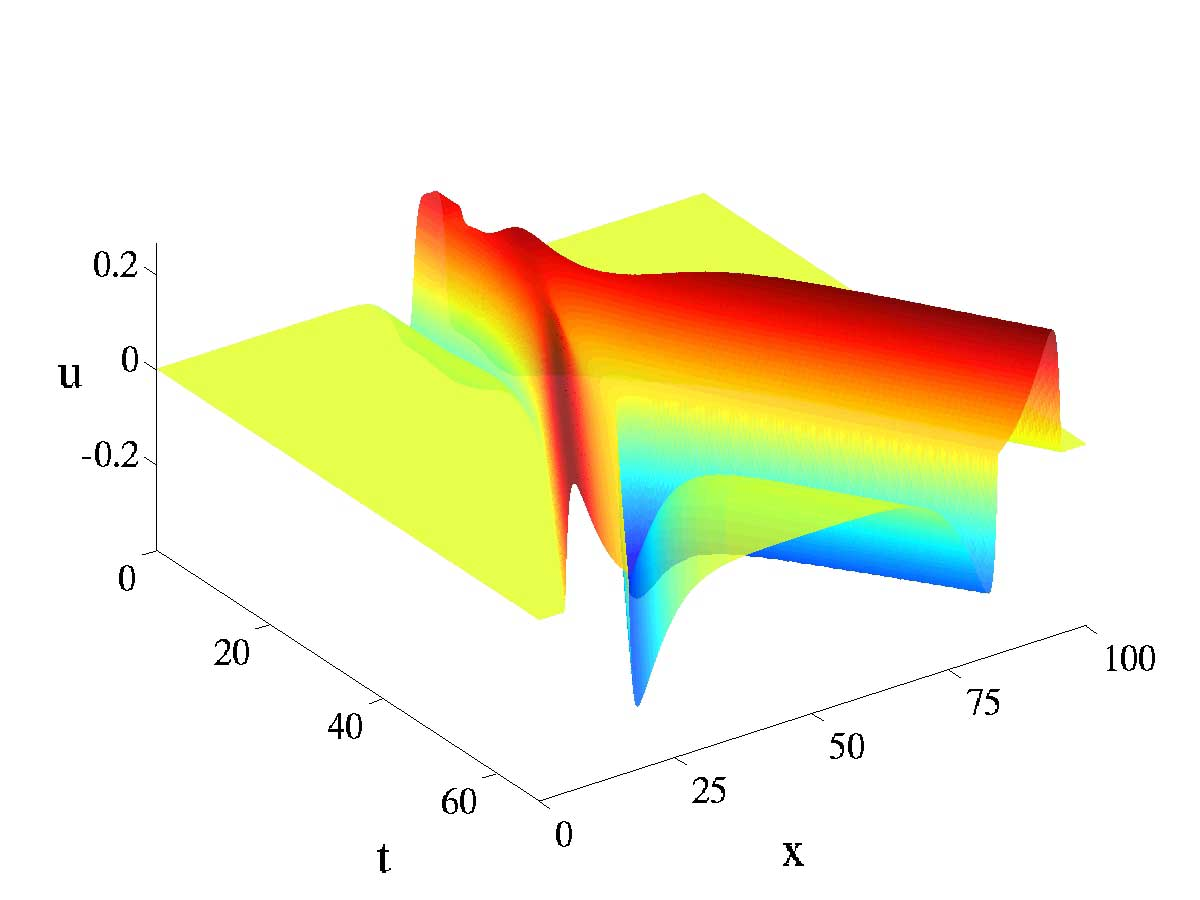Figure 5.7. A dimple bump shedding a pair of pulses.

S Coombes | Center for Mathematical Medicine | School of Mathematical Sciences | University of Nottingham

This Page: `http://www.maths.nott.ac.uk/personal/sc/evans/index.html`
Valid HTML(4.0 Transitional) // S Coombes Mar 30th 2004 `stephen.coombes@nottingham.ac.uk`